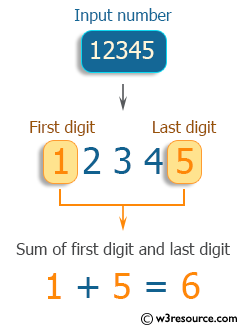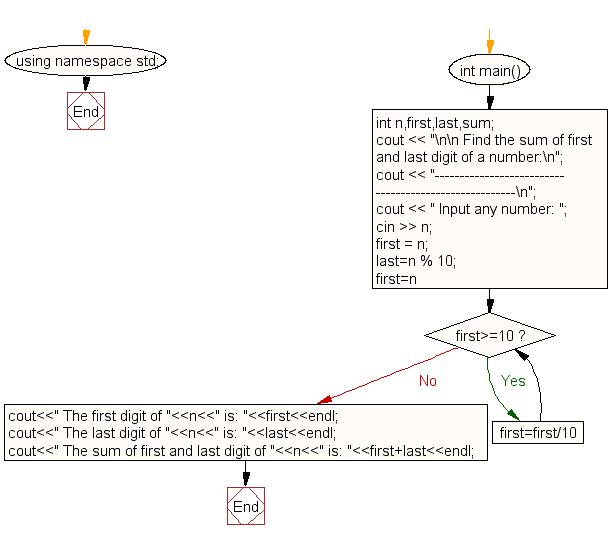﻿ C++ : Find the sum of first and last digit of a number

# C++ Exercises: Find the sum of first and last digit of a number

## C++ For Loop: Exercise-57 with Solution

Write a program in C++ to find the sum of the first and last digits of a number.

Pictorial Presentation:Sample Solution:

C++ Code :

``````#include <iostream>
using namespace std;

int main()
{
int n,first,last,sum;
cout << "\n\n Find the sum of first and last digit of a number:\n";
cout << "------------------------------------------------------\n";
cout << " Input any number: ";
cin >> n;
first = n;
last=n % 10;
for(first=n;first>=10;first=first/10);
cout<<" The first digit of "<<n<<" is: "<<first<<endl;
cout<<" The last digit of "<<n<<" is: "<<last<<endl;
cout<<" The sum of first and last digit of "<<n<<" is: "<<first+last<<endl;
}
``````

Sample Output:

``` Find the sum of first and last digit of a number:
------------------------------------------------------
Input any number: 12345
The first digit of 12345 is: 1
The last digit of 12345 is: 5
The sum of first and last digit of 12345 is: 6
```

Flowchart:C++ Code Editor: